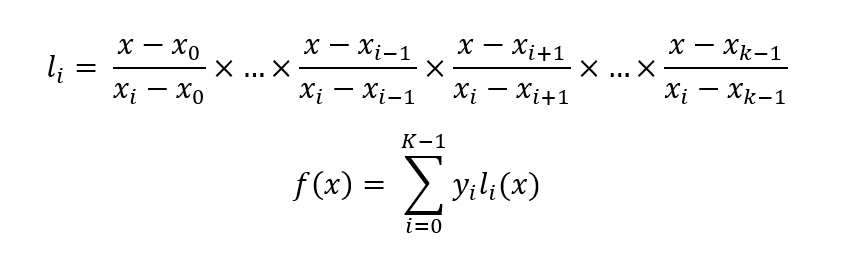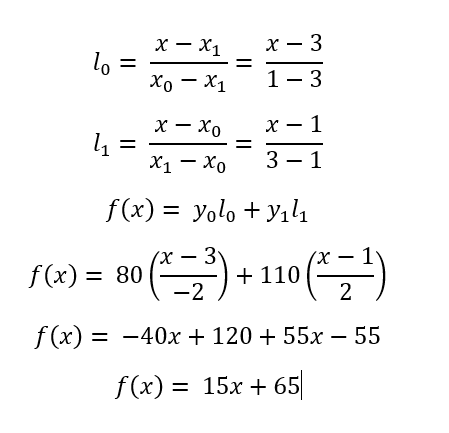# Shamir’s Secret Sharing Algorithm | Cryptography

Cryptography is a technique of securing information and communications through the use of codes so that only those person for whom the information is intended can understand it and process it. Thus preventing unauthorized access to information. The prefix “crypt” means “hidden” and suffix graphy means “writing”. In this article, a type of cryptographic technique, Shamir’s secret sharing algorithm is discussed.

Shamir’s Secret Sharing Algorithm: Shamir’s Secret Sharing is an algorithm in cryptography created by Adi Shamir. The main aim of this algorithm is to divide secret that needs to be encrypted into various unique parts.

• Let’s say S is the secret that we wish to encode.
• It is divided into N parts: S1, S2, S3, …., Sn.
• After dividing it, a number K is chosen by the user in order to decrypt the parts and find the original secret.
• It is chosen in such a way that if we know less than K parts, then we will not be able to find the secret S (i.e.) the secret S can not be reconstructed with (K – 1) parts or fewer.
• If we know K or more parts from S1, S2, S3, …., Sn, then we can compute/reconstructed our secret code S easily. This is conventionally called (K, N) threshold scheme.

Approach: The main idea behind the Shamir’s Secret Sharing Algorithm lies behind the concept that for the given K points we can find a polynomial equation with the degree (K – 1).

Example:

• For the given two points, (x1, y1) and (x2, y2) we can find a linear polynomial ax + by = c.
• Similarly, for the given three points, we can find a quadratic polynomial ax2 + bx + cy = d.

So, the idea is to build a polynomial with the degree (K – 1) such that the constant term is the secret code and the remaining numbers are random and this constant term can be found by using any K points out of N points generated from this polynomial by using Legrange’s Basis Polynomial.

For example: Let the secret code S = 65, N = 4, K = 2.

1. Initially, in order to encrypt the secret code, we build a polynomial of degree (K – 1).
2. Therefore, let the polynomial be y = a + bx. Here, the constant part ‘a’ is our secret code.
3. Let b be any random number, say b = 15.
4. Therefore, for this polynomial y = 65 + 15x, we generate N = 4 points from it.
5. Let those 4 points be (1, 80), (2, 95), (3, 110), (4, 125). Clearly, we can generate the initial polynomial from any two of these 4 points and in the resulting polynomial, the constant term a is the required secret code.

In order to reconstruct the given polynomial back, the Lagrange basis Polynomial is used.

The main concept behind the Lagrange polynomial is to form the Lagrange’s identities first and the summation of these identities give us the required function which we need to find from the given points. The following equations show how to compute them:For example, let’s say we wish to compute the given equation by using K = 2 points from the selected four points- (1, 80), (3, 110). The following illustration shows the step by step solution for this:Below is the implementation of the above approach:

 `// C++ implementation of shamir’s ` `// secret sharing algorithm ` ` `  `#include ` `using` `namespace` `std; ` ` `  `// Function to calculate the value ` `// of y ` `// y = poly + x*poly + x^2*poly + ... ` `int` `calculate_Y(``int` `x, vector<``int``>& poly) ` `{ ` `    ``// Initializing y ` `    ``int` `y = 0; ` `    ``int` `temp = 1; ` ` `  `    ``// Iterating through the array ` `    ``for` `(``auto` `coeff : poly) { ` ` `  `        ``// Computing the value of y ` `        ``y = (y + (coeff * temp)); ` `        ``temp = (temp * x); ` `    ``} ` `    ``return` `y; ` `} ` ` `  `// Function to perform the secret ` `// sharing algorithm and encode the ` `// given secret ` `void` `secret_sharing(``int` `S, vector >& points, ` `                    ``int` `N, ``int` `K) ` `{ ` `    ``// A vector to store the polynomial ` `    ``// cofficient of K-1 degree ` `    ``vector<``int``> poly(K); ` ` `  `    ``// Randomly choose K - 1 numbers but ` `    ``// not zero and poly is the secret ` `    ``// create polynomial for this ` ` `  `    ``poly = S; ` ` `  `    ``for` `(``int` `j = 1; j < K; ++j) { ` `        ``int` `p = 0; ` `        ``while` `(p == 0) ` ` `  `            ``// To keep the random values ` `            ``// in range not too high ` `            ``// we are taking mod with a ` `            ``// prime number around 1000 ` `            ``p = (``rand``() % 997); ` ` `  `        ``// This is to ensure we did not ` `        ``// create a polynomial consisting ` `        ``// of zeroes. ` `        ``poly[j] = p; ` `    ``} ` ` `  `    ``// Generating N points from the ` `    ``// polynomial we created ` `    ``for` `(``int` `j = 1; j <= N; ++j) { ` `        ``int` `x = j; ` `        ``int` `y = calculate_Y(x, poly); ` ` `  `        ``// Points created on sharing ` `        ``points[j - 1] = { x, y }; ` `    ``} ` `} ` ` `  `// This structure is used for fraction ` `// part handling multiplication ` `// and addition of fractiontion ` `struct` `fraction { ` `    ``int` `num, den; ` ` `  `    ``// A fraction consists of a ` `    ``// numerator and a denominator ` `    ``fraction(``int` `n, ``int` `d) ` `    ``{ ` `        ``num = n, den = d; ` `    ``} ` ` `  `    ``// If the fraction is not ` `    ``// in its reduced form ` `    ``// reduce it by dividing ` `    ``// them with their GCD ` `    ``void` `reduce_fraction(fraction& f) ` `    ``{ ` `        ``int` `gcd = __gcd(f.num, f.den); ` `        ``f.num /= gcd, f.den /= gcd; ` `    ``} ` ` `  `    ``// Performing multiplication on the ` `    ``// fraction ` `    ``fraction operator*(fraction f) ` `    ``{ ` `        ``fraction temp(num * f.num, den * f.den); ` `        ``reduce_fraction(temp); ` `        ``return` `temp; ` `    ``} ` ` `  `    ``// Performing addition on the ` `    ``// fraction ` `    ``fraction operator+(fraction f) ` `    ``{ ` `        ``fraction temp(num * f.den + den * f.num, ` `                      ``den * f.den); ` ` `  `        ``reduce_fraction(temp); ` `        ``return` `temp; ` `    ``} ` `}; ` ` `  `// Function to generate the secret ` `// back from the given points ` `// This function will use Lagrange Basis Polynomial ` `// Instead of finding the complete Polynomial ` `// We only required the poly as our secret code, ` `// thus we can get rid of x terms ` `int` `Generate_Secret(``int` `x[], ``int` `y[], ``int` `M) ` `{ ` ` `  `    ``fraction ans(0, 1); ` ` `  `    ``// Loop to iterate through the given ` `    ``// points ` `    ``for` `(``int` `i = 0; i < M; ++i) { ` ` `  `        ``// Initializing the fraction ` `        ``fraction l(y[i], 1); ` `        ``for` `(``int` `j = 0; j < M; ++j) { ` ` `  `            ``// Computing the lagrange terms ` `            ``if` `(i != j) { ` `                ``fraction temp(-x[j], x[i] - x[j]); ` `                ``l = l * temp; ` `            ``} ` `        ``} ` `        ``ans = ans + l; ` `    ``} ` ` `  `    ``// Return the secret ` `    ``return` `ans.num; ` `} ` ` `  `// Function to encode and decode the ` `// given secret by using the above ` `// defined functions ` `void` `operation(``int` `S, ``int` `N, ``int` `K) ` `{ ` ` `  `    ``// Vector to store the points ` `    ``vector > points(N); ` ` `  `    ``// Sharing of secret Code in N parts ` `    ``secret_sharing(S, points, N, K); ` ` `  `    ``cout << ``"Secret is divided to "` `<< N ` `         ``<< ``" Parts - "` `<< endl; ` ` `  `    ``for` `(``int` `i = 0; i < N; ++i) { ` `        ``cout << points[i].first << ``" "` `             ``<< points[i].second << endl; ` `    ``} ` ` `  `    ``cout << ``"We can generate Secret from any of "` `         ``<< K << ``" Parts"` `<< endl; ` ` `  `    ``// Input any M points from these  ` `    ``// to get back our secret code. ` `    ``int` `M = 2; ` ` `  `    ``// M can be greater than or equal to threshold but ` `    ``// for this example we are taking for threshold ` `    ``if` `(M < K) { ` `        ``cout << ``"Points are less than threshold "` `             ``<< K << ``" Points Required"` `<< endl; ` `    ``} ` ` `  `    ``int``* x = ``new` `int``[M]; ` `    ``int``* y = ``new` `int``[M]; ` ` `  `    ``// Input M points you will get the secret ` `    ``// Let these points are first M points from ` `    ``// the N points which we shared above ` `    ``// We can take any M points ` ` `  `    ``for` `(``int` `i = 0; i < M; ++i) { ` `        ``x[i] = points[i].first; ` `        ``y[i] = points[i].second; ` `    ``} ` ` `  `    ``// Get back our result again. ` `    ``cout << ``"Our Secret Code is : "` `         ``<< Generate_Secret(x, y, M) << endl; ` `} ` ` `  `// Driver Code ` `int` `main() ` `{ ` `    ``int` `S = 65;  ` `    ``int` `N = 4;  ` `    ``int` `K = 2;  ` ` `  `    ``operation(S, N, K); ` `    ``return` `0; ` `} `

Output:

```Secret is divided to 4 Parts -
1 602
2 1139
3 1676
4 2213
We can generate Secret from any of 2 Parts
Our Secret Code is : 65
```

Reference: Shamir Secret Sharing

Attention reader! Don’t stop learning now. Get hold of all the important CS Theory concepts for SDE interviews with the CS Theory Course at a student-friendly price and become industry ready.

My Personal Notes arrow_drop_upCheck out this Author's contributed articles.

If you like GeeksforGeeks and would like to contribute, you can also write an article using contribute.geeksforgeeks.org or mail your article to contribute@geeksforgeeks.org. See your article appearing on the GeeksforGeeks main page and help other Geeks.

Please Improve this article if you find anything incorrect by clicking on the "Improve Article" button below.